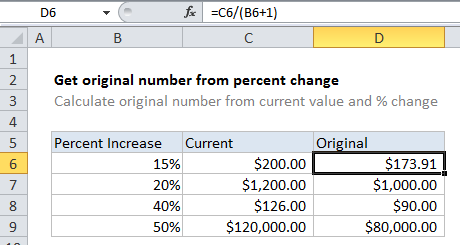## Excel Office

Excel How Tos, Tutorials, Tips & Tricks, Shortcuts

# How to get original number from percent change in Excel

To calculate the original number based on current value and known percentage change, you can use a simple formula that divides the current value by the percent plus 1.

Worked Example:   How To Create Budget in Excel

## Formula

`=current/(percent+1)`## Explanation

In the example, the active cell contains this formula:

`=C6/(B6+1)`

In this case, Excel first calculates the result of B6 + 1, then divides C6 by the result:

```=C6/(B6+1)
=200/1.15
=173.913```

### Rounded result

In the example shown, values in column D are using the currency format, which displays 2 decimal places automatically. If you want to force the result to be rounded to 2 decimal places, just wrap in the ROUND function:

`=ROUND(C6/(B6+1),2)`
Worked Example:   Understanding Named Range, Named Constant, Name Manager in Excel Formulas